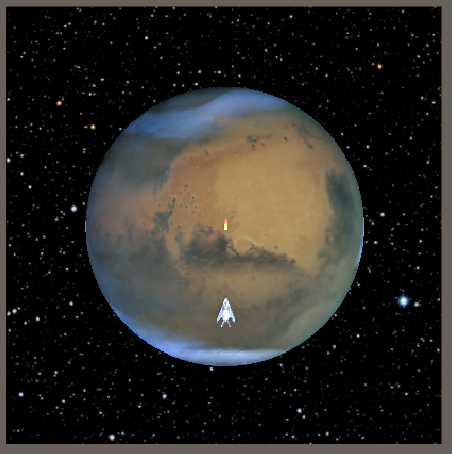# Rocket Game: Rocket did not fly! Why?,Problem about making a Rocket game: Rocket did not fly! Why?

I would like to ask a question for my rocket game:
PROBLEM:
My rocket did not fly, it stayed as a static picture in one place.
Every time the airplane shot a rocket clone, and it just stayed at where I pressed the key, it never fly.

I followed the tutorial book about how to make a simple airplane and rocket game in Unity 3D.
A 2D style game, but the objects beside from the background are all in 3D.

I would like to ask is there any problem with the script below? It is a C#script attached to the object rocket. I have made it a Prefab, and is already attached to the airplane.

-----( C#script attached to the object rocket)--------------------------------------------------------------------------
using System.Collections;
using System.Collections.Generic;
using UnityEngine;

public class Rocket : MonoBehaviour
{

``````public float m_speed = 10;

public float m_liveTime = 1;

public float m_power = 1.0f;

protected Transform m_trasform;

// Use this for initialization
void Start()
{

m_trasform = this.transform;
}

// Update is called once per frame
void Update()
{

m_liveTime -= Time.deltaTime;
if (m_liveTime <= 0)
Destroy(this.gameObject);

m_trasform.Translate(new Vector3(0, 0, -m_speed * Time.deltaTime));
}
``````

}

--------------( C#script attached to the object airplane)--------------------------------------
public class Player : MonoBehaviour
{
public float m_speed = 3;
public Transform m_rocket;
// Start is called before the first frame update
protected Transform m_transform;
void Start()
{
m_transform = this.transform;

``````}

// Update is called once per frame
void Update()
{
float movev = 0;
float moveh = 0;
if(Input.GetKey(KeyCode.UpArrow))
{
movev -= m_speed * Time.deltaTime;
}
if(Input.GetKey(KeyCode.DownArrow))
{
movev += m_speed * Time.deltaTime;
}
if(Input.GetKey(KeyCode.LeftArrow))
{
moveh += m_speed * Time.deltaTime;
}
if(Input.GetKey(KeyCode.RightArrow))
{
moveh -= m_speed * Time.deltaTime;
}
this.m_transform.Translate(new Vector3(moveh, 0, movev));

if (Input.GetKey(KeyCode.Space) || Input.GetMouseButton(0))
{
Instantiate(m_rocket, m_transform.position, m_transform.rotation);
}
}
``````

},I would like to ask a question for my rocket game:
PROBLEM:
My rocket did not fly, it stayed as a static picture in one place.
Every time the airplane shot a rocket clone, and it just stayed at where I pressed the key, it never fly.

I followed the tutorial book about how to make a simple airplane and rocket game in Unity 3D.
A 2D style game, but the objects beside from the background are all in 3D.

I would like to ask is there any problem with the script below? It is a C#script attached to the object rocket. I have made it a Prefab, and is already attached to the airplane.

-----( C#script attached to the object rocket)--------------------------------------------------------------------------
using System.Collections;
using System.Collections.Generic;
using UnityEngine;

public class Rocket : MonoBehaviour
{

``````public float m_speed = 10;

public float m_liveTime = 1;

public float m_power = 1.0f;

protected Transform m_trasform;

// Use this for initialization
void Start()
{

m_trasform = this.transform;
}

// Update is called once per frame
void Update()
{

m_liveTime -= Time.deltaTime;
if (m_liveTime <= 0)
Destroy(this.gameObject);

m_trasform.Translate(new Vector3(0, 0, -m_speed * Time.deltaTime));
}
``````

}

--------------( C#script attached to the object airplane)--------------------------------------
public class Player : MonoBehaviour
{
public float m_speed = 3;
public Transform m_rocket;
// Start is called before the first frame update
protected Transform m_transform;
void Start()
{
m_transform = this.transform;

``````}

// Update is called once per frame
void Update()
{
float movev = 0;
float moveh = 0;
if(Input.GetKey(KeyCode.UpArrow))
{
movev -= m_speed * Time.deltaTime;
}
if(Input.GetKey(KeyCode.DownArrow))
{
movev += m_speed * Time.deltaTime;
}
if(Input.GetKey(KeyCode.LeftArrow))
{
moveh += m_speed * Time.deltaTime;
}
if(Input.GetKey(KeyCode.RightArrow))
{
moveh -= m_speed * Time.deltaTime;
}
this.m_transform.Translate(new Vector3(moveh, 0, movev));

if (Input.GetKey(KeyCode.Space) || Input.GetMouseButton(0))
{
Instantiate(m_rocket, m_transform.position, m_transform.rotation);
}
}
``````

}So a way of fixing it could be:

``````public class Rocket : MonoBehaviour
{
public float m_speed = 10;
public float m_liveTime = 1;
public float m_power = 1.0f;
protected Transform m_trasform;

// Use this for initialization
void Start()
{
m_trasform = this.transform;
StartCoroutine(MoveRocket());
}

IEnumerator MoveRocket()
{
float endTime = Time.time + m_liveTime;
while (Time.time < endTime)
{
// Move the object forward along its z axis m_speed units/second.
// Maybe you should use Vector3.up instead but in your code it moves in the Z axis so
m_trasform.Translate(Vector3.forward * m_speed * Time.deltaTime);

yield return new WaitForEndOfFrame();
// we have to use this wait time bc Unity sometimes gets stuck in while loops
// You can declare a new WaitForSeconds at the top for better performance,
// but this looks smoother while moving
}

DestroyRocket();

yield return null;   // This is a statement needed by the coroutine to kinda know it ended.
}

// Use a different method to destroy it just in case
// you want to destroy it in the future under other conditions like a collision
void DestroyRocket()
{
Destroy(this.gameObject);
}
}
``````

We have moved the *moving* code to its own coroutine instead of using the Update method because of two reasons: first, the Update method receives a call every frame even if the GameObject is inactive (if I'm not wrong) so using a different method or coroutine is more performant. Second, coroutines are the way to go for those events that should run for a time and you want to avoid them from interfering with other code functionality . You can [learn more about them in the documentation].

I imagine you already know this but the *While* loop emulates the functionality of the Update method, and runs the code within it every frame. This way, it will update the transform of the game object and move it according to the speed you've set.

The call to Start the Coroutine should not be on Start but on it own public method (like SpawnRocket or whatever) to be called from the Fire event in the Player class, but I left it this way for simplicity shake.

As a side note, when you feel ready to do so, I encourage you to learn about object pooling for the rockets' instantiation.

Hope this helps. Happy coding